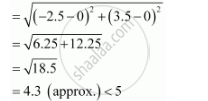CBSE (Commerce) Class 11CBSE
Share

Does the Point (–2.5, 3.5) Lie Inside, Outside Or on the Circle X2 + Y2 = 25? - CBSE (Commerce) Class 11 - Mathematics

Question

Does the point (–2.5, 3.5) lie inside, outside or on the circle x2 + y2 = 25?

Solution

The equation of the given circle is x2 + y2 = 25.

x2 + y2 = 25

⇒ (– 0)2 + (y – 0)2 = 52, which is of the form (x – h)2 + (y – k)2 = r2, where h = 0, k = 0, and r = 5.

∴Centre = (0, 0) and radius = 5

Distance between point (–2.5, 3.5) and centre (0, 0)Since the distance between point (–2.5, 3.5) and centre (0, 0) of the circle is less than the radius of the circle, point (–2.5, 3.5) lies inside the circle.

Is there an error in this question or solution?

Video TutorialsVIEW ALL 

Solution Does the Point (–2.5, 3.5) Lie Inside, Outside Or on the Circle X2 + Y2 = 25? Concept: Concept of Circle.
S